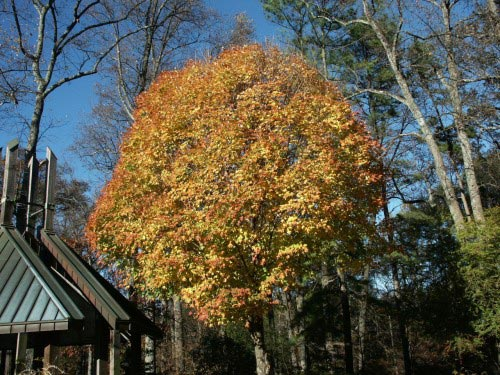## Tree Leaves – Calculating the Number## Tree Leaves – Calculating the Number

Q: My house is surrounded by very large, beautiful trees. I feel like I have raked a million leaves – and they aren’t even all down yet! Is there a way to calculate the approximate number of leaves on a tree? Between the leaves and the acorns, fall is quite a chore!

A: Forester Dr. Kim Coder says that a very rough estimate can be gotten by measuring the area (in square feet) beneath the crown of a tree and multiplying by 4. This gives you the estimated total leaf surface area of the tree in square feet. Next, estimate how many individual leaves it would take to cover one square foot. Multiply that result by the total leaf surface, which gives you an approximation of the number of leaves on that tree.

Example: the sweetgum in my front yard has a crown 30 feet wide. The area under it is approximately 700 square feet. Multiplied by 4, I see that the tree has 2,800 square feet of leaf area. I’ll guess that 8 sweetgum leaves would cover one square foot. Multiplying 8 times 2,800 reveals that the tree has close to 22,400 leaves.

A big oak tree might have a crown 50 feet wide. In that case, one could estimate the tree has 63,000 leaves. Necessarily, this is a rough guess because the crown shape of the tree is not taken into account (some trees are dome-shaped, some are tall and narrow, etc). However, this quick math should give you something to think about as you rake the last of the leaves from your lawn this week.